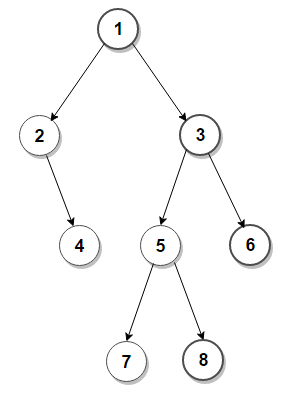# Print Right View of a Binary Tree

Given a binary tree, write an efficient algorithm to print right view of given binary tree.

For example, the right view of given binary tree is 1, 3, 6, 8### 1. Iterative Implementation using Queue –

In iterative version, we perform level order traversal of the tree. We can modify level order traversal to maintain nodes in current level. Then if current node is last node of current level, we print it.

C++ implementation –

The time complexity of above solution is O(n) and auxiliary space used by the program is O(n).

### 2. Recursive implementation using Hashing –

We can also solve this problem by using Hashing. The idea is to traverse the tree in preorder fashion and pass level information in function arguments. For every node encountered, we insert the node and level information into the map. Finally when all nodes are processed, we traverse the map and print the right view.

C++ implementation –

The time complexity of above solution is O(nlog(n)) and auxiliary space used by the program is O(n).

### Recursive implementation (using Preorder traversal) –

We can also solve this problem by using constant space and in linear time. The idea is to traverse the tree in reverse preorder fashion (visit right subtree before left subtree) and maintain maximum level visited so far. If current level is more than maximum level visited so far, then the current node is the last node of current level and we print it and also update last level to current level.

C++ implementation –

The time complexity of above solution is O(n) and need O(h) extra space for the call stack where h is the height of the tree.

Exercise: Print Left View of a Binary Tree(1 votes, average: 5.00 out of 5)Loading...